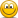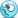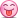# first week with lc

#### nasterun17

##### Newbie
Hello community,

wow what a great cheat. The customisation is so nice, i can change my settings in so many ways. I really love this. I played 6000h clean and I was good. With the cheat i do a high skill jump its feels so great.
Yeah I have little problems. An example is the Smooth aim, I don´t see differents between 5 smooth and 20 smooth. I am dump? maybe one of you can me explain this.
Next problem I have, is a second Crosshair in my normaly Crosshair, I only see this if I hold a p250, CZ or Duals.
I set the Option Crosshair=0 but the Problem still here.
At all I really like this Cheat and 1000x Thank You to you jim.

sincerly phil

PS: I came from Germany and I had a "E" in my English exameYes my English sucks

#### Sui7

##### I'm on Gear Bois
Hello community,

wow what a great cheat. The customisation is so nice, i can change my settings in so many ways. I really love this. I played 6000h clean and I was good. With the cheat i do a high skill jump its feels so great.
Yeah I have little problems. An example is the Smooth aim, I don´t see differents between 5 smooth and 20 smooth. I am dump? maybe one of you can me explain this.
Next problem I have, is a second Crosshair in my normaly Crosshair, I only see this if I hold a p250, CZ or Duals.
I set the Option Crosshair=0 but the Problem still here.
At all I really like this Cheat and 1000x Thank You to you jim.

sincerly phil

PS: I came from Germany and I had a "E" in my English exameYes my English sucks

Great to hear you're liking it. Could you maybe post your config here or pm it to me. So that I could take a look at why you're having the crosshair problem. Don't worry about your english it's understandable and can always be improved on.

Last edited:
•nasterun17

#### ToX

##### Retired Staff
Eligible for Pro League Beta
Thanks for your feedback, could you please tell me what super smooth are you using?
Also are you using m_rawinout 0? What sensitivity did you set?

Wysłane z iPhone za pomocą Tapatalk Pro

•nasterun17

#### nasterun17

##### Newbie
my Settings:
m_rawinput 0
sens: 1.06
windows: 6

1920x1080p

startopt. : -console -high -refresh 144 -tickrate 128 -exec autoexec +cl_cmdrate 128 -rate 786432 -nod3dex -nod3dex1 -noborder -sw

[SETTINGS]
;
;-ESP-
;
wall=0
wall2=0
dlight=0
decay=1
autointensity=1
intensity=0
dteam=1
crosshair=0
esp=0
box=0
boxsize=50
boxmulti=2
visesp=1
dis=0
hp=1
name=0
xesp=0
seq=0
index=0
pixel=0
pixelr=85
pixelg=107
pixelb=47
pixela=240
teamesp=0
disp=0
dispx=20
dispy=400
entity=0
noflash=0.20
weapon=0
listx=1750
listy=425
listesp=2
;
;-REGULAR AIMBOT SETTINGS-
;
aimbot=1
setcursortype=0
totalbones=3
cleandraw=1
smoothaim=8.00
multibone=2
bone=8
bone1=6
bone2=4
aimheight=0.00
aimright=0.00
fov=1.50
teamaim=1
aimdraw=0
aimkey=6
aimbutton=06
vischeck=1
aimclose=2
cdelta=3.00
shoot=0
rcsfov=25
aimtime=0.00
recoil=1.00
recoilx=1.00
recoilkey=1
recoiltype=6
nohop=1
recoilafter=0
ignorejumping=1
closefov=10.00
closefovdist=0
;
;-WEAPONCONFIG OPTION SETTINGS-
;
weaponconfig=1
weaponaimkey=1
weapontriggercfg=1
weapontriggerkey=0
flickshot=0
flickbutton=0x45
ammomgmt=1
;-TRIGGERBOT-
triggerbot=2
triggerdelay=200
triggerkey=4
triggerfov=0.00
triggerbones=0
triggerdraw=0
triggerbutton=4
triggerbone=4
triggerbone0=4
triggerbone1=5
triggerbone2=6
triggerbone3=0
triggerbone4=1
triggerbone5=2
triggerbone6=3
triggerbone7=8
triggerbone8=35
triggervischeck=2
triggerrandomdelay=1
triggermindelay=100
;
;-MISC
;
antiscreenshot=1
engcheck=1
neww2s=0
nofont=0
font=Verdana
fontsize=16
crosswidth=1
entdist=250
bhop=0
bhopkey=0x56
bhopmaxdelay=140
bhopmindelay=70
panickey=0x75
;
;-WEAPONCONFIGS - aimkey 1 is mouse1, aimkey 5 is mouse5
;
pistol_aimbot=1
pistol_aimkey=6
pistol_fov=1.75
pistol_rcsfov=0
pistol_aimright=0.00
pistol_aimheight=0.00
pistol_smoothaim=8.00
pistol_supersmooth=7
pistol_multibone=0
pistol_bone=8
pistol_bone1=0
pistol_bone2=0
pistol_recoil=0.00
pistol_recoilx=0.00
pistol_crosshair=0
pistol_triggerbot=2
pistol_triggerbones=0
pistol_nohop=1
pistol_aimtime=450.00
pistol_randomspot=1
;
deagle_aimbot=1
deagle_aimkey=6
deagle_fov=2.00
deagle_rcsfov=0
deagle_aimright=0.00
deagle_aimheight=0.00
deagle_smoothaim=7.00
deagle_supersmooth=4
deagle_multibone=0
deagle_bone=8
deagle_bone1=0
deagle_bone2=0
deagle_recoil=0.00
deagle_recoilx=0.00
deagle_crosshair=0
deagle_triggerbot=2
deagle_triggerbones=0
deagle_nohop=1
deagle_aimtime=450.00
degale_randomspot=1
;
sniper_aimbot=1
sniper_aimkey=6
sniper_fov=10.00
sniper_rcsfov=0
sniper_smoothaim=7.00
sniper_supersmooth=6
sniper_multibone=2
sniper_bone=6
sniper_bone1=8
sniper_bone2=4
sniper_aimheight=0.00
sniper_recoil=0.00
sniper_recoilx=0.00
sniper_crosshair=0
sniper_triggerbot=2
sniper_aimright=0.00
sniper_triggerbones=0
sniper_nohop=1
sniper_aimtime=450.00
sniper_randomspot=2
;
autosniper_aimbot=1
autosniper_aimkey=6
autosniper_fov=4.00
autosniper_rcsfov=20
autosniper_aimright=0.00
autosniper_aimheight=0.00
autosniper_smoothaim=5.00
autosniper_supersmooth=8
autosniper_multibone=2
autosniper_bone=4
autosniper_bone1=6
autosniper_bone2=5
autosniper_recoil=1.00
autosniper_recoilx=1.00
autosniper_crosshair=1
autosniper_triggerbot=2
autosniper_triggerbones=0
autosniper_nohop=0
autosniper_aimtime=0.00
autosniper_randomspot=2
;
rifle_aimbot=1
rifle_aimkey=6
rifle_fov=1.50
rifle_rcsfov=25
rifle_aimright=0.00
rifle_aimheight=0.00
rifle_smoothaim=10.00
rifle_supersmooth=6
rifle_multibone=2
rifle_bone=8
rifle_bone1=6
rifle_bone2=4
rifle_recoil=1.60
rifle_recoilx=6.00
rifle_crosshair=0
rifle_triggerbot=2
rifle_triggerbones=0
rifle_nohop=0
rifle_aimtime=300.00
rifle_randomspot=1
;
smg_aimbot=1
smg_aimkey=1
smg_fov=1.50
smg_rcsfov=25
smg_aimright=0.00
smg_aimheight=0.00
smg_smoothaim=7.00
smg_supersmooth=7
smg_multibone=1
smg_bone=5
smg_bone1=6
smg_bone2=5
smg_recoil=1.00
smg_recoilx=1.00
smg_crosshair=0
smg_triggerbot=2
smg_triggerbones=0
smg_nohop=1
smg_aimtime=400.00
amg_randomspot=1
;
shotgun_aimbot=1
shotgun_aimkey=1
shotgun_fov=4.00
shotgun_rcsfov=0
shotgun_aimright=0.00
shotgun_aimheight=0.00
shotgun_smoothaim=4.00
shotgun_supersmooth=6
shotgun_multibone=2
shotgun_bone=4
shotgun_bone1=6
shotgun_bone2=5
shotgun_recoil=0.00
shotgun_recoilx=0.00
shotgun_crosshair=0
shotgun_triggerbot=2
shotgun_triggerbones=0
shotgun_nohop=1
shotgun_aimtime=1500.00
shotgun_randomspot=3
;
pistolhp_aimbot=1
pistolhp_triggerbot=1
pistolhp_bone=6
pistolhp_bone1=5
pistolhp_bone2=4
pistolhp_bone3=3
pistolhp_bone4=2
pistolhp_smoothaim=5.00
pistolhp_fov=3.00
pistolhp_multibone=1
pistolhp_aimheight=1.00
pistolhp_aimright=1.00
pistolhp_aimkey=3
pistolhp_recoil=1.00
pistolhp_recoilx=1.00
pistolhp_triggerbones=3
pistolhp_crosshair=1
pistolhp_supersmooth=8
pistolhp_rcsfov=35
pistolhp_nohop=0
pistolhp_aimtime=0.00
pistolhp_shoot=0
pistolhp_randomspot=2
;
knife_aimbot=0
knife_triggerbot=0
;
pistol_closefovscoped=0
pistolhp_closefovscoped=0
deagle_closefovscoped=0
sniper_closefovscoped=0
autosniper_closefovscoped=0
rifle_closefovscoped=0
smg_closefovscoped=0
shotgun_closefovscoped=0
pistol_recoilafter=1
pistol_triggerkey=0
pistolhp_recoilafter=0
pistolhp_triggerkey=0
deagle_recoilafter=0
deagle_triggerkey=0
sniper_recoilafter=0
sniper_triggerkey=0
autosniper_recoilafter=0
autosniper_triggerkey=0
rifle_recoilafter=1
rifle_triggerkey=0
smg_recoilafter=0
smg_triggerkey=0
shotgun_recoilafter=0
shotgun_triggerkey=0
;
pistol_closefov=0.00
pistol_closefovdist=0
pistol_triggerdelay=0
pistol_triggerrandomdelay=0
pistol_triggermindelay=0
pistol_tfov=0.00
pistolhp_closefov=1.00
pistolhp_closefovdist=0
pistolhp_triggerdelay=0
pistolhp_triggerrandomdelay=0
pistolhp_triggermindelay=0
pistolhp_tfov=1.00
;
deagle_closefov=2.00
deagle_closefovdist=0
deagle_triggerdelay=200
deagle_triggerrandomdelay=1
deagle_triggermindelay=150
deagle_tfov=0.00
;
sniper_closefov=14.00
sniper_closefovdist=10
sniper_triggerdelay=180
sniper_triggerrandomdelay=1
sniper_triggermindelay=120
sniper_tfov=1.00
;
autosniper_closefov=5.00
autosniper_closefovdist=0
autosniper_triggerdelay=0
autosniper_triggerrandomdelay=0
autosniper_triggermindelay=0
autosniper_tfov=0.00
;
rifle_closefov=7.00
rifle_closefovdist=18
rifle_triggerdelay=180
rifle_triggerrandomdelay=1
rifle_triggermindelay=120
rifle_tfov=1.00
;
smg_closefov=2.00
smg_closefovdist=0
smg_triggerdelay=180
smg_triggerrandomdelay=1
smg_triggermindelay=120
smg_tfov=1.00
;
shotgun_closefov=4.00
shotgun_closefovdist=0
shotgun_triggerdelay=200
shotgun_triggerrandomdelay=1
shotgun_triggermindelay=100
shotgun_tfov=1.00
;
pistol_aimonshoot=0
pistol_triggerbone0=4
pistol_triggerbone1=7
pistol_triggerbone2=13
pistol_triggerbone3=2
pistol_triggerbone4=1
pistol_triggerbone5=34
pistol_triggerbone6=35
pistol_triggerbone7=33
pistol_triggerbone8=32
pistolhp_aimonshoot=0
pistolhp_triggerbone0=5
pistolhp_triggerbone1=6
pistolhp_triggerbone2=4
pistolhp_triggerbone3=5
pistolhp_triggerbone4=5
pistolhp_triggerbone5=5
pistolhp_triggerbone6=5
pistolhp_triggerbone7=5
pistolhp_triggerbone8=5
deagle_aimonshoot=0
deagle_triggerbone0=6
deagle_triggerbone1=5
deagle_triggerbone2=4
deagle_triggerbone3=3
deagle_triggerbone4=5
deagle_triggerbone5=5
deagle_triggerbone6=5
deagle_triggerbone7=5
deagle_triggerbone8=5
sniper_aimonshoot=0
sniper_triggerbone0=4
sniper_triggerbone1=6
sniper_triggerbone2=5
sniper_triggerbone3=2
sniper_triggerbone4=1
sniper_triggerbone5=34
sniper_triggerbone6=35
sniper_triggerbone7=33
sniper_triggerbone8=32
autosniper_aimonshoot=0
autosniper_triggerbone0=5
autosniper_triggerbone1=5
autosniper_triggerbone2=5
autosniper_triggerbone3=5
autosniper_triggerbone4=5
autosniper_triggerbone5=5
autosniper_triggerbone6=5
autosniper_triggerbone7=5
autosniper_triggerbone8=5
rifle_aimonshoot=0
rifle_triggerbone0=4
rifle_triggerbone1=3
rifle_triggerbone2=2
rifle_triggerbone3=6
rifle_triggerbone4=5
rifle_triggerbone5=6
rifle_triggerbone6=5
rifle_triggerbone7=5
rifle_triggerbone8=5
smg_aimonshoot=0
smg_triggerbone0=4
smg_triggerbone1=3
smg_triggerbone2=2
smg_triggerbone3=5
smg_triggerbone4=5
smg_triggerbone5=5
smg_triggerbone6=5
smg_triggerbone7=5
smg_triggerbone8=5
shotgun_aimonshoot=0
shotgun_triggerbone0=5
shotgun_triggerbone1=5
shotgun_triggerbone2=5
shotgun_triggerbone3=5
shotgun_triggerbone4=5
shotgun_triggerbone5=5
shotgun_triggerbone6=5
shotgun_triggerbone7=5
shotgun_triggerbone8=5
;
;-EXTRA BONES - change totalbone value to include these, more can be added in the same format-
;
bone3=1
bone4=2
bone5=3
pistol_bone3=1
pistol_bone4=2
pistol_bone5=3
deagle_bone3=1
deagle_bone4=2
deagle_bone5=3
sniper_bone3=1
sniper_bone4=2
sniper_bone5=3
autosniper_bone3=1
autosniper_bone4=2
autosniper_bone5=3
rifle_bone3=1
rifle_bone4=2
rifle_bone5=3
smg_bone3=1
smg_bone4=2
smg_bone5=3
shotgun_bone3=1
shotgun_bone4=2
shotgun_bone5=3
virtualkeypress=0
sendcmdafter=1
recoilcross=1
dynamicfov=1
softaim=1
supersmooth=1
randomspot=0
pistol_shoot=0
pistol_crosswidth=1
pistol_sendcmdafter=1
pistol_dynamicfov=1
pistol_recoilcross=1
pistol_softaim=1
pistolhp_crosswidth=1
pistolhp_sendcmdafter=1
pistolhp_dynamicfov=1
pistolhp_recoilcross=1
pistolhp_softaim=1
deagle_shoot=0
deagle_randomspot=0
deagle_crosswidth=1
deagle_sendcmdafter=1
deagle_dynamicfov=1
deagle_recoilcross=1
deagle_softaim=1
sniper_shoot=0
sniper_crosswidth=1
sniper_sendcmdafter=1
sniper_dynamicfov=1
sniper_recoilcross=1
sniper_softaim=1
autosniper_shoot=0
autosniper_crosswidth=1
autosniper_sendcmdafter=1
autosniper_dynamicfov=1
autosniper_recoilcross=1
autosniper_softaim=1
rifle_shoot=0
rifle_crosswidth=1
rifle_sendcmdafter=1
rifle_dynamicfov=1
rifle_recoilcross=1
rifle_softaim=1
smg_shoot=0
smg_randomspot=0
smg_crosswidth=1
smg_sendcmdafter=1
smg_dynamicfov=1
smg_recoilcross=1
smg_softaim=1
shotgun_shoot=0
shotgun_crosswidth=1
shotgun_sendcmdafter=1
shotgun_dynamicfov=1
shotgun_recoilcross=1
shotgun_softaim=1
knife_aimkey=3
rifle_m4_aimbot=1
rifle_m4_triggerbot=1
rifle_m4_aimkey=3
rifle_m4_bone=1
rifle_m4_bone1=1
rifle_m4_bone2=1
rifle_m4_smoothaim=1.00
rifle_m4_fov=1.00
rifle_m4_multibone=1
rifle_m4_aimheight=0.00
rifle_m4_aimright=0.00
rifle_m4_recoil=1.00
rifle_m4_recoilx=1.00
rifle_m4_triggerbones=0
rifle_m4_crosshair=1
rifle_m4_supersmooth=8
rifle_m4_rcsfov=35
rifle_m4_nohop=0
rifle_m4_aimtime=0.00
rifle_m4_shoot=0
rifle_m4_randomspot=0
rifle_m4_crosswidth=1
rifle_m4_sendcmdafter=1
rifle_m4_dynamicfov=1
rifle_m4_recoilcross=1
rifle_m4_softaim=1
rifle_m4_closefov=1.00
rifle_m4_closefovdist=0
rifle_m4_aimonshoot=0
rifle_m4_closefovscoped=0
rifle_m4_recoilafter=0
rifle_m4_triggerdelay=0
rifle_m4_triggerkey=0
rifle_m4_triggerrandomdelay=0
rifle_m4_triggermindelay=0
rifle_m4_tfov=1.25
rifle_m4_triggerbone0=5
rifle_m4_triggerbone1=5
rifle_m4_triggerbone2=5
rifle_m4_triggerbone3=5
rifle_m4_triggerbone4=5
rifle_m4_triggerbone5=5
rifle_m4_triggerbone6=5
rifle_m4_triggerbone7=5
rifle_m4_triggerbone8=5
rifle_ak_aimbot=1
rifle_ak_triggerbot=1
rifle_ak_aimkey=3
rifle_ak_bone=1
rifle_ak_bone1=1
rifle_ak_bone2=1
rifle_ak_smoothaim=1.00
rifle_ak_fov=1.00
rifle_ak_multibone=1
rifle_ak_aimheight=0.00
rifle_ak_aimright=0.00
rifle_ak_recoil=1.00
rifle_ak_recoilx=1.00
rifle_ak_triggerbones=0
rifle_ak_crosshair=1
rifle_ak_supersmooth=8
rifle_ak_rcsfov=35
rifle_ak_nohop=0
rifle_ak_aimtime=0.00
rifle_ak_shoot=0
rifle_ak_randomspot=0
rifle_ak_crosswidth=1
rifle_ak_sendcmdafter=1
rifle_ak_dynamicfov=1
rifle_ak_recoilcross=1
rifle_ak_softaim=1
rifle_ak_closefov=1.00
rifle_ak_closefovdist=0
rifle_ak_aimonshoot=0
rifle_ak_closefovscoped=0
rifle_ak_recoilafter=0
rifle_ak_triggerdelay=0
rifle_ak_triggerkey=0
rifle_ak_triggerrandomdelay=0
rifle_ak_triggermindelay=0
rifle_ak_tfov=1.25
rifle_ak_triggerbone0=5
rifle_ak_triggerbone1=5
rifle_ak_triggerbone2=5
rifle_ak_triggerbone3=5
rifle_ak_triggerbone4=5
rifle_ak_triggerbone5=5
rifle_ak_triggerbone6=5
rifle_ak_triggerbone7=5
rifle_ak_triggerbone8=5
sniper_awp_aimbot=1
sniper_awp_triggerbot=1
sniper_awp_aimkey=3
sniper_awp_bone=1
sniper_awp_bone1=1
sniper_awp_bone2=1
sniper_awp_smoothaim=1.00
sniper_awp_fov=1.00
sniper_awp_multibone=1
sniper_awp_aimheight=0.00
sniper_awp_aimright=0.00
sniper_awp_recoil=1.00
sniper_awp_recoilx=1.00
sniper_awp_triggerbones=0
sniper_awp_crosshair=1
sniper_awp_supersmooth=8
sniper_awp_rcsfov=35
sniper_awp_nohop=0
sniper_awp_aimtime=0.00
sniper_awp_shoot=0
sniper_awp_randomspot=0
sniper_awp_crosswidth=1
sniper_awp_sendcmdafter=1
sniper_awp_dynamicfov=1
sniper_awp_recoilcross=1
sniper_awp_softaim=1
sniper_awp_closefov=1.00
sniper_awp_closefovdist=0
sniper_awp_aimonshoot=0
sniper_awp_closefovscoped=0
sniper_awp_recoilafter=0
sniper_awp_triggerdelay=0
sniper_awp_triggerkey=0
sniper_awp_triggerrandomdelay=0
sniper_awp_triggermindelay=0
sniper_awp_tfov=1.25
sniper_awp_triggerbone0=5
sniper_awp_triggerbone1=5
sniper_awp_triggerbone2=5
sniper_awp_triggerbone3=5
sniper_awp_triggerbone4=5
sniper_awp_triggerbone5=5
sniper_awp_triggerbone6=5
sniper_awp_triggerbone7=5
sniper_awp_triggerbone8=5
sniper_scout_aimbot=1
sniper_scout_triggerbot=1
sniper_scout_aimkey=3
sniper_scout_bone=1
sniper_scout_bone1=1
sniper_scout_bone2=1
sniper_scout_smoothaim=1.00
sniper_scout_fov=1.00
sniper_scout_multibone=1
sniper_scout_aimheight=0.00
sniper_scout_aimright=0.00
sniper_scout_recoil=1.00
sniper_scout_recoilx=1.00
sniper_scout_triggerbones=0
sniper_scout_crosshair=1
sniper_scout_supersmooth=8
sniper_scout_rcsfov=35
sniper_scout_nohop=0
sniper_scout_aimtime=0.00
sniper_scout_shoot=0
sniper_scout_randomspot=0
sniper_scout_crosswidth=1
sniper_scout_sendcmdafter=1
sniper_scout_dynamicfov=1
sniper_scout_recoilcross=1
sniper_scout_softaim=1
sniper_scout_closefov=1.00
sniper_scout_closefovdist=0
sniper_scout_aimonshoot=0
sniper_scout_closefovscoped=0
sniper_scout_recoilafter=0
sniper_scout_triggerdelay=0
sniper_scout_triggerkey=0
sniper_scout_triggerrandomdelay=0
sniper_scout_triggermindelay=0
sniper_scout_tfov=1.25
sniper_scout_triggerbone0=5
sniper_scout_triggerbone1=5
sniper_scout_triggerbone2=5
sniper_scout_triggerbone3=5
sniper_scout_triggerbone4=5
sniper_scout_triggerbone5=5
sniper_scout_triggerbone6=5
sniper_scout_triggerbone7=5
sniper_scout_triggerbone8=5
pistol_glock_aimbot=1
pistol_glock_triggerbot=1
pistol_glock_aimkey=3
pistol_glock_bone=1
pistol_glock_bone1=1
pistol_glock_bone2=1
pistol_glock_smoothaim=1.00
pistol_glock_fov=1.00
pistol_glock_multibone=1
pistol_glock_aimheight=0.00
pistol_glock_aimright=0.00
pistol_glock_recoil=1.00
pistol_glock_recoilx=1.00
pistol_glock_triggerbones=0
pistol_glock_crosshair=1
pistol_glock_supersmooth=8
pistol_glock_rcsfov=35
pistol_glock_nohop=0
pistol_glock_aimtime=0.00
pistol_glock_shoot=0
pistol_glock_randomspot=0
pistol_glock_crosswidth=1
pistol_glock_sendcmdafter=1
pistol_glock_dynamicfov=1
pistol_glock_recoilcross=1
pistol_glock_softaim=1
pistol_glock_closefov=1.00
pistol_glock_closefovdist=0
pistol_glock_aimonshoot=0
pistol_glock_closefovscoped=0
pistol_glock_recoilafter=0
pistol_glock_triggerdelay=0
pistol_glock_triggerkey=0
pistol_glock_triggerrandomdelay=0
pistol_glock_triggermindelay=0
pistol_glock_tfov=1.25
pistol_glock_triggerbone0=5
pistol_glock_triggerbone1=5
pistol_glock_triggerbone2=5
pistol_glock_triggerbone3=5
pistol_glock_triggerbone4=5
pistol_glock_triggerbone5=5
pistol_glock_triggerbone6=5
pistol_glock_triggerbone7=5
pistol_glock_triggerbone8=5

Last edited:

#### Dexter Morgan

##### Global Elite
Welcomeglad that you are enjoying itSent from my ONEPLUS A5010 using Tapatalk

•nasterun17

#### Sui7

##### I'm on Gear Bois
my Settings:
m_rawinput 0
sens: 1.06
windows: 6

1920x1080p

startopt. : -console -high -refresh 144 -tickrate 128 -exec autoexec +cl_cmdrate 128 -rate 786432 -nod3dex -nod3dex1 -noborder -sw

[SETTINGS]
;
;-ESP-
;
wall=0
wall2=0
dlight=0
decay=1
autointensity=1
intensity=0
dteam=1
crosshair=0
esp=0
box=0
boxsize=50
boxmulti=2
visesp=1
dis=0
hp=1
name=0
xesp=0
seq=0
index=0
pixel=0
pixelr=85
pixelg=107
pixelb=47
pixela=240
teamesp=0
disp=0
dispx=20
dispy=400
entity=0
noflash=0.20
weapon=0
listx=1750
listy=425
listesp=2
;
;-REGULAR AIMBOT SETTINGS-
;
aimbot=1
setcursortype=0
totalbones=3
cleandraw=1
smoothaim=8.00
multibone=2
bone=8
bone1=6
bone2=4
aimheight=0.00
aimright=0.00
fov=1.50
teamaim=1
aimdraw=0
aimkey=6
aimbutton=06
vischeck=1
aimclose=2
cdelta=3.00
shoot=0
rcsfov=25
aimtime=0.00
recoil=1.00
recoilx=1.00
recoilkey=1
recoiltype=6
nohop=1
recoilafter=0
ignorejumping=1
closefov=10.00
closefovdist=0
;
;-WEAPONCONFIG OPTION SETTINGS-
;
weaponconfig=1
weaponaimkey=1
weapontriggercfg=1
weapontriggerkey=0
flickshot=0
flickbutton=0x45
ammomgmt=1
;-TRIGGERBOT-
triggerbot=2
triggerdelay=200
triggerkey=4
triggerfov=0.00
triggerbones=0
triggerdraw=0
triggerbutton=4
triggerbone=4
triggerbone0=4
triggerbone1=5
triggerbone2=6
triggerbone3=0
triggerbone4=1
triggerbone5=2
triggerbone6=3
triggerbone7=8
triggerbone8=35
triggervischeck=2
triggerrandomdelay=1
triggermindelay=100
;
;-MISC
;
antiscreenshot=1
engcheck=1
neww2s=0
nofont=0
font=Verdana
fontsize=16
crosswidth=1
entdist=250
bhop=0
bhopkey=0x56
bhopmaxdelay=140
bhopmindelay=70
panickey=0x75
;
;-WEAPONCONFIGS - aimkey 1 is mouse1, aimkey 5 is mouse5
;
pistol_aimbot=1
pistol_aimkey=6
pistol_fov=1.75
pistol_rcsfov=0
pistol_aimright=0.00
pistol_aimheight=0.00
pistol_smoothaim=8.00
pistol_supersmooth=7
pistol_multibone=0
pistol_bone=8
pistol_bone1=0
pistol_bone2=0
pistol_recoil=0.00
pistol_recoilx=0.00
pistol_crosshair=0
pistol_triggerbot=2
pistol_triggerbones=0
pistol_nohop=1
pistol_aimtime=450.00
pistol_randomspot=1
;
deagle_aimbot=1
deagle_aimkey=6
deagle_fov=2.00
deagle_rcsfov=0
deagle_aimright=0.00
deagle_aimheight=0.00
deagle_smoothaim=7.00
deagle_supersmooth=4
deagle_multibone=0
deagle_bone=8
deagle_bone1=0
deagle_bone2=0
deagle_recoil=0.00
deagle_recoilx=0.00
deagle_crosshair=0
deagle_triggerbot=2
deagle_triggerbones=0
deagle_nohop=1
deagle_aimtime=450.00
degale_randomspot=1
;
sniper_aimbot=1
sniper_aimkey=6
sniper_fov=10.00
sniper_rcsfov=0
sniper_smoothaim=7.00
sniper_supersmooth=6
sniper_multibone=2
sniper_bone=6
sniper_bone1=8
sniper_bone2=4
sniper_aimheight=0.00
sniper_recoil=0.00
sniper_recoilx=0.00
sniper_crosshair=0
sniper_triggerbot=2
sniper_aimright=0.00
sniper_triggerbones=0
sniper_nohop=1
sniper_aimtime=450.00
sniper_randomspot=2
;
autosniper_aimbot=1
autosniper_aimkey=6
autosniper_fov=4.00
autosniper_rcsfov=20
autosniper_aimright=0.00
autosniper_aimheight=0.00
autosniper_smoothaim=5.00
autosniper_supersmooth=8
autosniper_multibone=2
autosniper_bone=4
autosniper_bone1=6
autosniper_bone2=5
autosniper_recoil=1.00
autosniper_recoilx=1.00
autosniper_crosshair=1
autosniper_triggerbot=2
autosniper_triggerbones=0
autosniper_nohop=0
autosniper_aimtime=0.00
autosniper_randomspot=2
;
rifle_aimbot=1
rifle_aimkey=6
rifle_fov=1.50
rifle_rcsfov=25
rifle_aimright=0.00
rifle_aimheight=0.00
rifle_smoothaim=10.00
rifle_supersmooth=6
rifle_multibone=2
rifle_bone=8
rifle_bone1=6
rifle_bone2=4
rifle_recoil=1.60
rifle_recoilx=6.00
rifle_crosshair=0
rifle_triggerbot=2
rifle_triggerbones=0
rifle_nohop=0
rifle_aimtime=300.00
rifle_randomspot=1
;
smg_aimbot=1
smg_aimkey=1
smg_fov=1.50
smg_rcsfov=25
smg_aimright=0.00
smg_aimheight=0.00
smg_smoothaim=7.00
smg_supersmooth=7
smg_multibone=1
smg_bone=5
smg_bone1=6
smg_bone2=5
smg_recoil=1.00
smg_recoilx=1.00
smg_crosshair=0
smg_triggerbot=2
smg_triggerbones=0
smg_nohop=1
smg_aimtime=400.00
amg_randomspot=1
;
shotgun_aimbot=1
shotgun_aimkey=1
shotgun_fov=4.00
shotgun_rcsfov=0
shotgun_aimright=0.00
shotgun_aimheight=0.00
shotgun_smoothaim=4.00
shotgun_supersmooth=6
shotgun_multibone=2
shotgun_bone=4
shotgun_bone1=6
shotgun_bone2=5
shotgun_recoil=0.00
shotgun_recoilx=0.00
shotgun_crosshair=0
shotgun_triggerbot=2
shotgun_triggerbones=0
shotgun_nohop=1
shotgun_aimtime=1500.00
shotgun_randomspot=3
;
pistolhp_aimbot=1
pistolhp_triggerbot=1
pistolhp_bone=6
pistolhp_bone1=5
pistolhp_bone2=4
pistolhp_bone3=3
pistolhp_bone4=2
pistolhp_smoothaim=5.00
pistolhp_fov=3.00
pistolhp_multibone=1
pistolhp_aimheight=1.00
pistolhp_aimright=1.00
pistolhp_aimkey=3
pistolhp_recoil=1.00
pistolhp_recoilx=1.00
pistolhp_triggerbones=3
pistolhp_crosshair=1
pistolhp_supersmooth=8
pistolhp_rcsfov=35
pistolhp_nohop=0
pistolhp_aimtime=0.00
pistolhp_shoot=0
pistolhp_randomspot=2
;
knife_aimbot=0
knife_triggerbot=0
;
pistol_closefovscoped=0
pistolhp_closefovscoped=0
deagle_closefovscoped=0
sniper_closefovscoped=0
autosniper_closefovscoped=0
rifle_closefovscoped=0
smg_closefovscoped=0
shotgun_closefovscoped=0
pistol_recoilafter=1
pistol_triggerkey=0
pistolhp_recoilafter=0
pistolhp_triggerkey=0
deagle_recoilafter=0
deagle_triggerkey=0
sniper_recoilafter=0
sniper_triggerkey=0
autosniper_recoilafter=0
autosniper_triggerkey=0
rifle_recoilafter=1
rifle_triggerkey=0
smg_recoilafter=0
smg_triggerkey=0
shotgun_recoilafter=0
shotgun_triggerkey=0
;
pistol_closefov=0.00
pistol_closefovdist=0
pistol_triggerdelay=0
pistol_triggerrandomdelay=0
pistol_triggermindelay=0
pistol_tfov=0.00
pistolhp_closefov=1.00
pistolhp_closefovdist=0
pistolhp_triggerdelay=0
pistolhp_triggerrandomdelay=0
pistolhp_triggermindelay=0
pistolhp_tfov=1.00
;
deagle_closefov=2.00
deagle_closefovdist=0
deagle_triggerdelay=200
deagle_triggerrandomdelay=1
deagle_triggermindelay=150
deagle_tfov=0.00
;
sniper_closefov=14.00
sniper_closefovdist=10
sniper_triggerdelay=180
sniper_triggerrandomdelay=1
sniper_triggermindelay=120
sniper_tfov=1.00
;
autosniper_closefov=5.00
autosniper_closefovdist=0
autosniper_triggerdelay=0
autosniper_triggerrandomdelay=0
autosniper_triggermindelay=0
autosniper_tfov=0.00
;
rifle_closefov=7.00
rifle_closefovdist=18
rifle_triggerdelay=180
rifle_triggerrandomdelay=1
rifle_triggermindelay=120
rifle_tfov=1.00
;
smg_closefov=2.00
smg_closefovdist=0
smg_triggerdelay=180
smg_triggerrandomdelay=1
smg_triggermindelay=120
smg_tfov=1.00
;
shotgun_closefov=4.00
shotgun_closefovdist=0
shotgun_triggerdelay=200
shotgun_triggerrandomdelay=1
shotgun_triggermindelay=100
shotgun_tfov=1.00
;
pistol_aimonshoot=0
pistol_triggerbone0=4
pistol_triggerbone1=7
pistol_triggerbone2=13
pistol_triggerbone3=2
pistol_triggerbone4=1
pistol_triggerbone5=34
pistol_triggerbone6=35
pistol_triggerbone7=33
pistol_triggerbone8=32
pistolhp_aimonshoot=0
pistolhp_triggerbone0=5
pistolhp_triggerbone1=6
pistolhp_triggerbone2=4
pistolhp_triggerbone3=5
pistolhp_triggerbone4=5
pistolhp_triggerbone5=5
pistolhp_triggerbone6=5
pistolhp_triggerbone7=5
pistolhp_triggerbone8=5
deagle_aimonshoot=0
deagle_triggerbone0=6
deagle_triggerbone1=5
deagle_triggerbone2=4
deagle_triggerbone3=3
deagle_triggerbone4=5
deagle_triggerbone5=5
deagle_triggerbone6=5
deagle_triggerbone7=5
deagle_triggerbone8=5
sniper_aimonshoot=0
sniper_triggerbone0=4
sniper_triggerbone1=6
sniper_triggerbone2=5
sniper_triggerbone3=2
sniper_triggerbone4=1
sniper_triggerbone5=34
sniper_triggerbone6=35
sniper_triggerbone7=33
sniper_triggerbone8=32
autosniper_aimonshoot=0
autosniper_triggerbone0=5
autosniper_triggerbone1=5
autosniper_triggerbone2=5
autosniper_triggerbone3=5
autosniper_triggerbone4=5
autosniper_triggerbone5=5
autosniper_triggerbone6=5
autosniper_triggerbone7=5
autosniper_triggerbone8=5
rifle_aimonshoot=0
rifle_triggerbone0=4
rifle_triggerbone1=3
rifle_triggerbone2=2
rifle_triggerbone3=6
rifle_triggerbone4=5
rifle_triggerbone5=6
rifle_triggerbone6=5
rifle_triggerbone7=5
rifle_triggerbone8=5
smg_aimonshoot=0
smg_triggerbone0=4
smg_triggerbone1=3
smg_triggerbone2=2
smg_triggerbone3=5
smg_triggerbone4=5
smg_triggerbone5=5
smg_triggerbone6=5
smg_triggerbone7=5
smg_triggerbone8=5
shotgun_aimonshoot=0
shotgun_triggerbone0=5
shotgun_triggerbone1=5
shotgun_triggerbone2=5
shotgun_triggerbone3=5
shotgun_triggerbone4=5
shotgun_triggerbone5=5
shotgun_triggerbone6=5
shotgun_triggerbone7=5
shotgun_triggerbone8=5
;
;-EXTRA BONES - change totalbone value to include these, more can be added in the same format-
;
bone3=1
bone4=2
bone5=3
pistol_bone3=1
pistol_bone4=2
pistol_bone5=3
deagle_bone3=1
deagle_bone4=2
deagle_bone5=3
sniper_bone3=1
sniper_bone4=2
sniper_bone5=3
autosniper_bone3=1
autosniper_bone4=2
autosniper_bone5=3
rifle_bone3=1
rifle_bone4=2
rifle_bone5=3
smg_bone3=1
smg_bone4=2
smg_bone5=3
shotgun_bone3=1
shotgun_bone4=2
shotgun_bone5=3
virtualkeypress=0
sendcmdafter=1
recoilcross=1
dynamicfov=1
softaim=1
supersmooth=1
randomspot=0
pistol_shoot=0
pistol_crosswidth=1
pistol_sendcmdafter=1
pistol_dynamicfov=1
pistol_recoilcross=1
pistol_softaim=1
pistolhp_crosswidth=1
pistolhp_sendcmdafter=1
pistolhp_dynamicfov=1
pistolhp_recoilcross=1
pistolhp_softaim=1
deagle_shoot=0
deagle_randomspot=0
deagle_crosswidth=1
deagle_sendcmdafter=1
deagle_dynamicfov=1
deagle_recoilcross=1
deagle_softaim=1
sniper_shoot=0
sniper_crosswidth=1
sniper_sendcmdafter=1
sniper_dynamicfov=1
sniper_recoilcross=1
sniper_softaim=1
autosniper_shoot=0
autosniper_crosswidth=1
autosniper_sendcmdafter=1
autosniper_dynamicfov=1
autosniper_recoilcross=1
autosniper_softaim=1
rifle_shoot=0
rifle_crosswidth=1
rifle_sendcmdafter=1
rifle_dynamicfov=1
rifle_recoilcross=1
rifle_softaim=1
smg_shoot=0
smg_randomspot=0
smg_crosswidth=1
smg_sendcmdafter=1
smg_dynamicfov=1
smg_recoilcross=1
smg_softaim=1
shotgun_shoot=0
shotgun_crosswidth=1
shotgun_sendcmdafter=1
shotgun_dynamicfov=1
shotgun_recoilcross=1
shotgun_softaim=1
knife_aimkey=3
rifle_m4_aimbot=1
rifle_m4_triggerbot=1
rifle_m4_aimkey=3
rifle_m4_bone=1
rifle_m4_bone1=1
rifle_m4_bone2=1
rifle_m4_smoothaim=1.00
rifle_m4_fov=1.00
rifle_m4_multibone=1
rifle_m4_aimheight=0.00
rifle_m4_aimright=0.00
rifle_m4_recoil=1.00
rifle_m4_recoilx=1.00
rifle_m4_triggerbones=0
rifle_m4_crosshair=1
rifle_m4_supersmooth=8
rifle_m4_rcsfov=35
rifle_m4_nohop=0
rifle_m4_aimtime=0.00
rifle_m4_shoot=0
rifle_m4_randomspot=0
rifle_m4_crosswidth=1
rifle_m4_sendcmdafter=1
rifle_m4_dynamicfov=1
rifle_m4_recoilcross=1
rifle_m4_softaim=1
rifle_m4_closefov=1.00
rifle_m4_closefovdist=0
rifle_m4_aimonshoot=0
rifle_m4_closefovscoped=0
rifle_m4_recoilafter=0
rifle_m4_triggerdelay=0
rifle_m4_triggerkey=0
rifle_m4_triggerrandomdelay=0
rifle_m4_triggermindelay=0
rifle_m4_tfov=1.25
rifle_m4_triggerbone0=5
rifle_m4_triggerbone1=5
rifle_m4_triggerbone2=5
rifle_m4_triggerbone3=5
rifle_m4_triggerbone4=5
rifle_m4_triggerbone5=5
rifle_m4_triggerbone6=5
rifle_m4_triggerbone7=5
rifle_m4_triggerbone8=5
rifle_ak_aimbot=1
rifle_ak_triggerbot=1
rifle_ak_aimkey=3
rifle_ak_bone=1
rifle_ak_bone1=1
rifle_ak_bone2=1
rifle_ak_smoothaim=1.00
rifle_ak_fov=1.00
rifle_ak_multibone=1
rifle_ak_aimheight=0.00
rifle_ak_aimright=0.00
rifle_ak_recoil=1.00
rifle_ak_recoilx=1.00
rifle_ak_triggerbones=0
rifle_ak_crosshair=1
rifle_ak_supersmooth=8
rifle_ak_rcsfov=35
rifle_ak_nohop=0
rifle_ak_aimtime=0.00
rifle_ak_shoot=0
rifle_ak_randomspot=0
rifle_ak_crosswidth=1
rifle_ak_sendcmdafter=1
rifle_ak_dynamicfov=1
rifle_ak_recoilcross=1
rifle_ak_softaim=1
rifle_ak_closefov=1.00
rifle_ak_closefovdist=0
rifle_ak_aimonshoot=0
rifle_ak_closefovscoped=0
rifle_ak_recoilafter=0
rifle_ak_triggerdelay=0
rifle_ak_triggerkey=0
rifle_ak_triggerrandomdelay=0
rifle_ak_triggermindelay=0
rifle_ak_tfov=1.25
rifle_ak_triggerbone0=5
rifle_ak_triggerbone1=5
rifle_ak_triggerbone2=5
rifle_ak_triggerbone3=5
rifle_ak_triggerbone4=5
rifle_ak_triggerbone5=5
rifle_ak_triggerbone6=5
rifle_ak_triggerbone7=5
rifle_ak_triggerbone8=5
sniper_awp_aimbot=1
sniper_awp_triggerbot=1
sniper_awp_aimkey=3
sniper_awp_bone=1
sniper_awp_bone1=1
sniper_awp_bone2=1
sniper_awp_smoothaim=1.00
sniper_awp_fov=1.00
sniper_awp_multibone=1
sniper_awp_aimheight=0.00
sniper_awp_aimright=0.00
sniper_awp_recoil=1.00
sniper_awp_recoilx=1.00
sniper_awp_triggerbones=0
sniper_awp_crosshair=1
sniper_awp_supersmooth=8
sniper_awp_rcsfov=35
sniper_awp_nohop=0
sniper_awp_aimtime=0.00
sniper_awp_shoot=0
sniper_awp_randomspot=0
sniper_awp_crosswidth=1
sniper_awp_sendcmdafter=1
sniper_awp_dynamicfov=1
sniper_awp_recoilcross=1
sniper_awp_softaim=1
sniper_awp_closefov=1.00
sniper_awp_closefovdist=0
sniper_awp_aimonshoot=0
sniper_awp_closefovscoped=0
sniper_awp_recoilafter=0
sniper_awp_triggerdelay=0
sniper_awp_triggerkey=0
sniper_awp_triggerrandomdelay=0
sniper_awp_triggermindelay=0
sniper_awp_tfov=1.25
sniper_awp_triggerbone0=5
sniper_awp_triggerbone1=5
sniper_awp_triggerbone2=5
sniper_awp_triggerbone3=5
sniper_awp_triggerbone4=5
sniper_awp_triggerbone5=5
sniper_awp_triggerbone6=5
sniper_awp_triggerbone7=5
sniper_awp_triggerbone8=5
sniper_scout_aimbot=1
sniper_scout_triggerbot=1
sniper_scout_aimkey=3
sniper_scout_bone=1
sniper_scout_bone1=1
sniper_scout_bone2=1
sniper_scout_smoothaim=1.00
sniper_scout_fov=1.00
sniper_scout_multibone=1
sniper_scout_aimheight=0.00
sniper_scout_aimright=0.00
sniper_scout_recoil=1.00
sniper_scout_recoilx=1.00
sniper_scout_triggerbones=0
sniper_scout_crosshair=1
sniper_scout_supersmooth=8
sniper_scout_rcsfov=35
sniper_scout_nohop=0
sniper_scout_aimtime=0.00
sniper_scout_shoot=0
sniper_scout_randomspot=0
sniper_scout_crosswidth=1
sniper_scout_sendcmdafter=1
sniper_scout_dynamicfov=1
sniper_scout_recoilcross=1
sniper_scout_softaim=1
sniper_scout_closefov=1.00
sniper_scout_closefovdist=0
sniper_scout_aimonshoot=0
sniper_scout_closefovscoped=0
sniper_scout_recoilafter=0
sniper_scout_triggerdelay=0
sniper_scout_triggerkey=0
sniper_scout_triggerrandomdelay=0
sniper_scout_triggermindelay=0
sniper_scout_tfov=1.25
sniper_scout_triggerbone0=5
sniper_scout_triggerbone1=5
sniper_scout_triggerbone2=5
sniper_scout_triggerbone3=5
sniper_scout_triggerbone4=5
sniper_scout_triggerbone5=5
sniper_scout_triggerbone6=5
sniper_scout_triggerbone7=5
sniper_scout_triggerbone8=5
pistol_glock_aimbot=1
pistol_glock_triggerbot=1
pistol_glock_aimkey=3
pistol_glock_bone=1
pistol_glock_bone1=1
pistol_glock_bone2=1
pistol_glock_smoothaim=1.00
pistol_glock_fov=1.00
pistol_glock_multibone=1
pistol_glock_aimheight=0.00
pistol_glock_aimright=0.00
pistol_glock_recoil=1.00
pistol_glock_recoilx=1.00
pistol_glock_triggerbones=0
pistol_glock_crosshair=1
pistol_glock_supersmooth=8
pistol_glock_rcsfov=35
pistol_glock_nohop=0
pistol_glock_aimtime=0.00
pistol_glock_shoot=0
pistol_glock_randomspot=0
pistol_glock_crosswidth=1
pistol_glock_sendcmdafter=1
pistol_glock_dynamicfov=1
pistol_glock_recoilcross=1
pistol_glock_softaim=1
pistol_glock_closefov=1.00
pistol_glock_closefovdist=0
pistol_glock_aimonshoot=0
pistol_glock_closefovscoped=0
pistol_glock_recoilafter=0
pistol_glock_triggerdelay=0
pistol_glock_triggerkey=0
pistol_glock_triggerrandomdelay=0
pistol_glock_triggermindelay=0
pistol_glock_tfov=1.25
pistol_glock_triggerbone0=5
pistol_glock_triggerbone1=5
pistol_glock_triggerbone2=5
pistol_glock_triggerbone3=5
pistol_glock_triggerbone4=5
pistol_glock_triggerbone5=5
pistol_glock_triggerbone6=5
pistol_glock_triggerbone7=5
pistol_glock_triggerbone8=5

Please remove auth. @ToX could you remove it for him?

Also heres the problem for the crosshair pistolhp_crosshair=1 if you change this to 0 you should be fine.

EDIT: @Aaron Could you remove this guys auth details as ToX isn't hereLast edited:

#### nasterun17

##### Newbie
@Sui7 what is "auth." ?

#### nasterun17

##### Newbie
Oh fuck iam so dump sorry

is done

#### Sui7

##### I'm on Gear Bois
Oh fuck iam so dump sorry

is done

No problem we all make mistakes. Did the pistolhp_crosshair=0 fix your problem?

•nasterun17

#### nasterun17

##### Newbie
@Sui7 thx it works perfectly

#### FD^GoD

##### Ex Helper
Hello community,

wow what a great cheat. The customisation is so nice, i can change my settings in so many ways. I really love this. I played 6000h clean and I was good. With the cheat i do a high skill jump its feels so great.
Yeah I have little problems. An example is the Smooth aim, I don´t see differents between 5 smooth and 20 smooth. I am dump? maybe one of you can me explain this.
Next problem I have, is a second Crosshair in my normaly Crosshair, I only see this if I hold a p250, CZ or Duals.
I set the Option Crosshair=0 but the Problem still here.
At all I really like this Cheat and 1000x Thank You to you jim.

sincerly phil

PS: I came from Germany and I had a "E" in my English exameYes my English sucks
Thx for you review bro. Your problem is probably from reason that you have added crosshair command in your default ESP or aimbot settings or you just activeted recoil crosshair .

•nasterun17

#### ParaNox1337

##### Silver II
Eligible for Pro League Beta
cevo will be owned by you#### Nattanoj

##### Newbie
yeah its a great cheat, i had a total 1+ year sub in this cheat in other account, i could own people so easy with this 2d pixel aimbot.

Its great, buying it soon again

#### fmr

##### Big Boss
Nice testimonial!